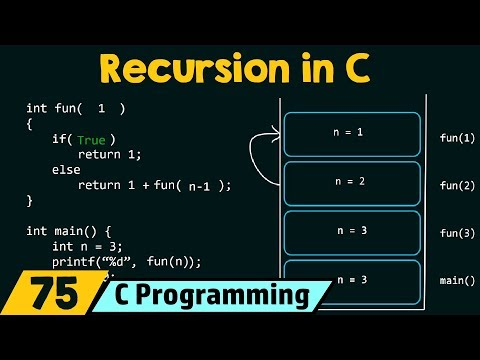A process in which function called itself directly or indirectly is called recursion in C, and that function is called recursive function. A recursive function can solve many problems like Fibonacci series, the factorial of a number and Towers of Hanoi (TOH), etc.

Let's understand it with the help of an example.

int function1 (int n)
{
function1 ( );
//code;
}

function1() is a recursive function that calls itself. If the termination condition is not inserted in the recursive function function1(), then stack overflow will occur. So, it is necessary to put a condition to avoid an infinite loop (avoid stack overflow).

int function1 (int n)
{
if (condition)
function1 ( );
//code;
}

### Direct recursion in C

When a function called itself is known as direct recursion in C.
{
//code
//code
}

### Indirect recursion in C

When a function called another recursive function, and the called function calling the call function is known as indirect recursion in C.
For example, add() function is known as an indirect recursive function because it calls sub() function and sub() function call add() function.

{
//code
sub( );
//code
}
void sub( )
{
//code
}

### How does recursion work?

If we are making a recursive function, then we should keep in mind that each call of the recursive function creates a new automatic set of variables.
Let's try to understand it with a  program. In the below program, the recursive function has been created to calculate factorial of a number. What is factorial? Factorial is a product of all positive number from 1 to n.

Factorial of a number can be,
n! = n*(n-1)!
For example, (4!) = 4 * (4-1)! = 4* (3!)
= 4*3*(3-1)!
= 4*3*(2!)
=4*3*2*(2-1)!
=4*3*2*(1)!
= 4*3*2*1
=24

Note 0! and 1! will be 1.

### Program to find out factorial of a given number.

#include <stdio.h>
int factorial(int num); //Declaration of factorial function
int main( )
{
int num = 5, output=0
if (num <0)
{
printf ("Factorial of a negative number is not possible");
}
else
{
output = factorial(num);
printf ("Factorial is %d\n", output);
}
return 0;
}

int factorial(int num)
{
if (num==0)
{
return 1;
}
else
{
return (num*factorial (num-1));
}
}

Output
Factorial is 120.

### Working on the above program

Desired number (num)= 5
factorial (5)=5*factorial (4)
factorial (4)= 4*factorial (3)
factorial (3)=3*factorial (2)
factorial (2)=2*factorial (1)
factorial (1)=1*factorial (0)

When num=0 then the recursion process stops and control returns to factorial(1). Now reverse process occurs, and function will return a value to the previous function calls.

So, the output will be, factorial (5) = 5*4*3*2*1 = 120

Recursion process has a lot of advantage and disadvantage. Some are mentioning here,

1. Recursion process helps us to makes the program simpler.
2. With the help of recursion, a lot of mathematical problems can be solved like calculate factorial of a number, generate Fibonacci series.
3. Recursive functions are convenient for recursively defined data structure like trees.

1. Recursion in C reduces the speed of a program because each time it creates a stack frame for the function call.
2. Recursion needs a lot of memory space.

Learn Recursion in CReviewed by Glory on 13:01 Rating: 5